List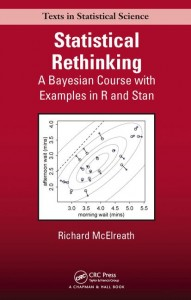Here I work through the practice questions in Chapter 6, “Overfitting, Regularization, and Information Criteria,” of Statistical Rethinking (McElreath, 2016). I do my best to use only approaches and functions discussed so far in the book, as well as to name objects consistently with how the book does. If you find any typos or mistakes in my answers, or if you have any relevant questions, please feel free to add a comment below.

Here is the chapter summary from page 205:

This chapter has been a marathon. It began with the problem of overfitting, a universal phenomenon by which models with more parameters fit a sample better, even when the additional parameters are meaningless. Two common tools were introduced to address overfitting: regularizing priors and information criteria. Regularizing priors reduce overfitting during estimation, and information criteria help estimate the degree of overfitting. Practical functions compare() and ensemble() in the rethinking package were introduced to help analyze collections of models fit to the same data. In the chapters to follow, these tools will be applied to both new and old data examples. In all cases, keep in mind that these tools are heuristic. They provide no guarantees. No statistical procedure will ever substitute for iterative scientific investigation.

# Setup
library(rethinking)
data(Howell1)
set.seed(6)


## Easy Difficulty

### Practice Question 6E1

State the three motivating criteria that define information entropy. Try to express each in your own words.

The motivating criteria are described on pages 177-178. In my own words, they are that a measure of uncertainty should (1) be measured on a continuous scale such that the spacing between adjacent values is consistent, (2) capture the size of the possibility space such that its value scales with the number of possible outcomes, and (3) be additive for independent events such that it does not matter how the events are divided. This last criterion is the most confusing, but is also very important. It states that dividing a possibility space into four events (e.g., rain/hot, rain/cold, shine/hot, shine/cold) provides the same amount of information as dividing this same possibility space into two sets of two events (e.g., rain-or-shine and hot-or-cold).

### Practice Question 6E2

Suppose a coin is weighted such that, when it is tossed and lands on a table, it comes up heads 70% of the time. What is the entropy of this coin?

Entropy is defined on page 178. We just need to plug in the appropriate probabilities.

$H(p) = -\sum_{i=1}^n p_i \log(p_i) = -(p_H \log(p_H)+p_T \log(p_T))$

p <- c(0.7, 1 - 0.7)
(H <- -sum(p * log(p)))

##  0.6108643


The entropy of this coin is 0.61.

### Practice Question 6E3

Suppose a four-sided die is loaded such that, when tossed onto a table, it shows “1” 20%, “2” 25%, “3” 25%, and “4” 30% of the time. What is the entropy of this die?

We can use the same approach as above (page 178).

p <- c(0.20, 0.25, 0.25, 0.30)
(H <- -sum(p * log(p)))

##  1.376227


The entropy of this die is 1.38.

### Practice Question 6E4

Suppose another four-sided die is loaded such that it never shows “4”. The other three sides show equally often. What is the entropy of this die?

We can use the same approach as above (page 178). The only complication is that we need to use L’Hopital’s rule and ignore the side that never shows (page 179).

p <- c(1/3, 1/3, 1/3)
(H <- -sum(p * log(p)))

##  1.098612


The entropy of the die is 1.10.

## Medium Difficulty

### Practice Question 6M1

Write down and compare the definitions of AIC, DIC, and WAIC. Which of these criteria is most general? Which assumptions are required to transform a more general criterion into a less general one?

AIC is defined as $$D_{train}+2p$$ where $$D_{train}$$ is the in-sample training deviance and $$p$$ is the number of free parameters estimated in the model (page 189).

DIC is defined as $$\bar{D}+p_D=\bar{D}+(\bar{D}+\hat{D})$$ where $$\bar{D}$$ is the average of the posterior distribution of deviance and $$\hat{D}$$ is the deviance calculated at the posterior mean (page 190).

WAIC is defined as $$-2(\mathrm{lppd}-p_{WAIC})=-2(\sum_{i=1}^N \log\Pr(y_i) – \sum_{i=1}^N V(y_i))$$ where $$\Pr(y_i)$$ is the average likelihood of observation $$i$$ in the training sample and $$V(y_i)$$ is the variance in log-likelihood for observation $$i$$ in the training sample (pages 191-192).

All three definitions involve two components: an estimate or analog of the in-sample training deviance (i.e., $$D_{train}$$ for AIC, $$\bar{D}$$ for DIC, and $$\mathrm{lppd}$$ for WAIC) and an estimate or analog for the number of free parameters estimated in the model (i.e., $$p$$ in AIC, $$p_D$$ in DIC, and $$p_{WAIC}$$ in WAIC).

WAIC is the most general, followed by DIC, and finally AIC (page 190). To move from WAIC to DIC, we must assume that the posterior distribution is approximately multivariate Gaussian. To move from DIC to AIC, we must further assume that the priors are flat or overwhelmed by the likelihood.

### Practice Question 6M2

Explain the difference between model selection and model averaging. What information is lost under model selection? What information is lost under model averaging?

Model selection is choosing to retain (e.g., use and interpret) the model with the lowest information criterion value and to discard all other models with higher values (page 195). This practice loses information about relative model accuracy contained in the differences among information criterion values; this is especially problematic when the selected model only outperforms its alternatives to a small degree. Model averaging is using Bayesian information criteria to construct a posterior predictive distribution (i.e., make predictions) that leverages the uncertainty in multiple models (page 196). This practice does not lose information on its own. However, when combined with undisclosed data dredging (page 205), it can lead to spurious findings (e.g., non-generalizable coincidence).

### Practice Question 6M3

When comparing models with an information criterion, why must all models be fit to exactly the same observations? What would happen to the information criterion values, if the models were fit to different numbers of observations? Perform some experiments, if you are not sure.

Information criteria are based on deviance, which is accrued over observations without being divided by the number of observations (page 182). Thus, it is a sum and not an average. So, all else being equal, a model with more observations will have a higher deviance and thus worse accuracy according to information criteria. It would be an unfair comparison to contrast models fit to different numbers of observations.

As an experiment, we can calculate WAIC for models fit to increasingly small subsamples of the same data. The information criteria should decrease alongside the sample size. In order to get a large sample to begin with, I will return to the Howell1 database from last chapter.

d <- Howell1[complete.cases(Howell1), ]
d_500 <- d[sample(1:nrow(d), size = 500, replace = FALSE), ]
d_400 <- d[sample(1:nrow(d), size = 400, replace = FALSE), ]
d_300 <- d[sample(1:nrow(d), size = 300, replace = FALSE), ]
m_500 <- map(
alist(
height ~ dnorm(mu, sigma),
mu <- a + b * log(weight)
),
data = d_500,
start = list(a = mean(d_500$height), b = 0, sigma = sd(d_500$height))
)
m_400 <- map(
alist(
height ~ dnorm(mu, sigma),
mu <- a + b * log(weight)
),
data = d_400,
start = list(a = mean(d_400$height), b = 0, sigma = sd(d_400$height))
)
m_300 <- map(
alist(
height ~ dnorm(mu, sigma),
mu <- a + b * log(weight)
),
data = d_300,
start = list(a = mean(d_300$height), b = 0, sigma = sd(d_300$height))
)
(model.compare <- compare(m_500, m_400, m_300))

## Warning in compare(m_500, m_400, m_300): Different numbers of observations found for at least two models.
## Information criteria only valid for comparing models fit to exactly same observations.
## Number of observations for each model:
## m_500 500
## m_400 400
## m_300 300

## Warning in waic_ptw1 - waic_ptw2: longer object length is not a multiple of
## shorter object length

## Warning in waic_ptw1 - waic_ptw2: longer object length is not a multiple of
## shorter object length

## Warning in waic_ptw1 - waic_ptw2: longer object length is not a multiple of
## shorter object length

##         WAIC pWAIC  dWAIC weight    SE   dSE
## m_300 1835.6   3.6    0.0      1 29.97    NA
## m_400 2457.5   3.3  621.9      0 31.90 44.00
## m_500 3071.2   3.2 1235.6      0 35.49 50.32


Indeed, the WAIC increased from the $$N=300$$ model to the $$N=400$$ model and again to the $$N=500$$ model. The compare() function also returns a warning about the number of observations being different.

### Practice Question 6M4

What happens to the effective number of parameters, as measured by DIC or WAIC, as a prior becomes more concentrated? Why? Perform some experiments, if you are not sure.

As the prior becomes more concentrated (e.g., as in a regularizing prior), the effective number of parameters decreases. In the case of DIC, this is because $$p_D$$ is a measure of how flexible the model is (page 191); with more concentrated priors, the model becomes less flexible (and therefore less prone to overfitting). In the case of WAIC, this is because $$p_{WAIC}$$ is a measure of the variance in the log-likelihood for each observation in the training sample (page 191); with more concentrated priors, the likelihood will become more concentrated as well and thus variance will decrease.

As an experiment to demonstrate this, we can calculate WAIC for two models of the same data that only differ in the concentration of their priors.

d <- Howell1[complete.cases(Howell1), ]
d$height.log <- log(d$height)
d$height.log.z <- (d$height.log - mean(d$height.log)) / sd(d$height.log)
d$weight.log <- log(d$weight)
d$weight.log.z <- (d$weight.log - mean(d$weight.log)) / sd(d$weight.log)
m_wide <- map(
alist(
height.log.z ~ dnorm(mu, sigma),
mu <- a + b * weight.log.z,
a ~ dnorm(0, 10),
b ~ dnorm(1, 10),
sigma ~ dunif(0, 10)
),
data = d
)
m_narrow <- map(
alist(
height.log.z ~ dnorm(mu, sigma),
mu <- a + b * weight.log.z,
a ~ dnorm(0, 0.10),
b ~ dnorm(1, 0.10),
sigma ~ dunif(0, 1)
),
data = d
)
WAIC(m_wide, refresh = 0)

##  -102.7533
## attr(,"lppd")
##  55.64182
## attr(,"pWAIC")
##  4.265166
## attr(,"se")
##  36.52922

WAIC(m_narrow, refresh = 0)

##  -102.7489
## attr(,"lppd")
##  55.60564
## attr(,"pWAIC")
##  4.231208
## attr(,"se")
##  36.47835


Indeed, the $$p_{WAIC}$$ decreases as the priors become more concentrated. However, note that the regularizing priors must be chosen carefully in order for this to occur (see 6M6).

### Practice Question 6M5

Provide an informal explanation of why informative priors reduce overfitting.

Informative priors reduce overfitting because they constrain the flexibility of the model; they make it less likely for extreme parameter values to be assigned high posterior probability. In simpler terms, informative priors reduce overfitting by forcing the model to learn less from the sample data.

### Practice Question 6M6

Provide an informal explanation of why overly informative priors result in underfitting.

Overly informative priors result in underfitting because they constrain the flexibility of the model too much; they make it less likely for “correct” parameter values to be assigned high posterior probability. In simpler terms, overly informative priors result in underfitting by preventing the model from learning enough from the sample data.

## Hard Difficulty

All practice problems to follow use the same data. Pull out the old Howell !Kung demography data and split it into two equally sized data frames. Here’s code to do it:

d <- Howell1

d$age <- (d$age - mean(d$age)) / sd(d$age)

set.seed(1000)

i <- sample(1:nrow(d), size = nrow(d) / 2)

d1 <- d[i, ]

d2 <- d[-i, ]

You now have two randomly formed data frames, each with 272 rows. The notion here is to use the cases in d1 to fit models and the cases in d2 to evaluate them. The set.seed() command just ensures that everyone works with the same randomly shuffled data.

Now let $$h_i$$ and $$x_i$$ be the height and centered age values, respectively, on row $$i$$. Fit the following models to the data in d1:

\begin{aligned} \mathcal{M}_1: h_i &\sim \mathrm{Normal}(\mu_i, \sigma) \\ \mu_i &= \alpha + \beta_1 x_i \\ \mathcal{M}_2: h_i &\sim \mathrm{Normal}(\mu_i, \sigma) \\ \mu_i &= \alpha + \beta_1 x_i + \beta_2 x_i^2 \\ \mathcal{M}_3: h_i &\sim \mathrm{Normal}(\mu_i, \sigma) \\ \mu_i &= \alpha + \beta_1 x_i + \beta_2 x_i^2 + \beta_3 x_i^3 \\ \mathcal{M}_4: h_i &\sim \mathrm{Normal}(\mu_i, \sigma) \\ \mu_i &= \alpha + \beta_1 x_i + \beta_2 x_i^2 + \beta_3 x_i^3 + \beta_4 x_i^4 \\ \mathcal{M}_5: h_i &\sim \mathrm{Normal}(\mu_i, \sigma) \\ \mu_i &= \alpha + \beta_1 x_i + \beta_2 x_i^2 + \beta_3 x_i^3 + \beta_4 x_i^4 + \beta_5 x_i^5 \\ \mathcal{M}_6: h_i &\sim \mathrm{Normal}(\mu_i, \sigma) \\ \mu_i &= \alpha + \beta_1 x_i + \beta_2 x_i^2 + \beta_3 x_i^3 + \beta_4 x_i^4 + \beta_5 x_i^5 + \beta_6 x_i^6 \end{aligned}

Use map() to fit these. Use weakly regularizing priors for all parameters.

Note that fitting all of these polynomials to the height-by-age relationship is not a good way to derive insight. It would be better to have a simpler approach that would allow for more insight, like perhaps a piecewise linear model. But the set of polynomial families above will serve to help you practice and understand model comparison and averaging.

### Practice Question 6H1

Compare the models above, using WAIC. Compare the model rankings, as well as the WAIC weights.

First let’s prep the data.

d <- Howell1
d$age <- (d$age - mean(d$age)) / sd(d$age)
set.seed(1000)
i <- sample(1:nrow(d), size = nrow(d) / 2)
d1 <- d[i, ]
d2 <- d[-i, ]


Now we can fit the models to d1 using map():

m1 <- map(
alist(
height ~ dnorm(mu, sigma),
mu <- a + b1 * age,
a ~ dnorm(140, 30),
b1 ~ dnorm(0, 50),
sigma ~ dnorm(30, 10)
),
data = d1
)
m2 <- map(
alist(
height ~ dnorm(mu, sigma),
mu <- a + b1 * age + b2 * I(age^2),
a ~ dnorm(140, 30),
b1 ~ dnorm(0, 50),
b2 ~ dnorm(0, 50),
sigma ~ dnorm(30, 10)
),
data = d1
)
m3 <- map(
alist(
height ~ dnorm(mu, sigma),
mu <- a + b1 * age + b2 * I(age^2) + b3 * I(age^3),
a ~ dnorm(140, 30),
b1 ~ dnorm(0, 50),
b2 ~ dnorm(0, 50),
b3 ~ dnorm(0, 50),
sigma ~ dnorm(30, 10)
),
data = d1
)
m4 <- map(
alist(
height ~ dnorm(mu, sigma),
mu <- a + b1 * age + b2 * I(age^2) + b3 * I(age^3) + b4 * I(age^4),
a ~ dnorm(140, 30),
b1 ~ dnorm(0, 50),
b2 ~ dnorm(0, 50),
b3 ~ dnorm(0, 50),
b4 ~ dnorm(0, 50),
sigma ~ dnorm(30, 10)
),
data = d1
)
m5 <- map(
alist(
height ~ dnorm(mu, sigma),
mu <- a + b1 * age + b2 * I(age^2) + b3 * I(age^3) + b4 * I(age^4) + b5 * I(age^5),
a ~ dnorm(140, 30),
b1 ~ dnorm(0, 50),
b2 ~ dnorm(0, 50),
b3 ~ dnorm(0, 50),
b4 ~ dnorm(0, 50),
b5 ~ dnorm(0, 50),
sigma ~ dnorm(30, 10)
),
data = d1
)
m6 <- map(
alist(
height ~ dnorm(mu, sigma),
mu <- a + b1 * age + b2 * I(age^2) + b3 * I(age^3) + b4 * I(age^4) + b5 * I(age^5) + b6 * I(age^6),
a ~ dnorm(140, 30),
b1 ~ dnorm(0, 50),
b2 ~ dnorm(0, 50),
b3 ~ dnorm(0, 50),
b4 ~ dnorm(0, 50),
b5 ~ dnorm(0, 50),
b6 ~ dnorm(0, 50),
sigma ~ dnorm(30, 10)
),
data = d1
)


Now let’s compare the models with WAIC:

(comparison <- compare(m1, m2, m3, m4, m5, m6, refresh = 0))

##      WAIC pWAIC dWAIC weight    SE   dSE
## m4 1926.0   5.6   0.0   0.56 25.30    NA
## m5 1927.5   6.4   1.4   0.28 25.16  1.07
## m6 1928.6   7.7   2.5   0.16 24.88  2.80
## m3 1952.3   5.4  26.2   0.00 24.03 10.73
## m2 2149.8   5.0 223.7   0.00 22.38 26.48
## m1 2395.4   3.4 469.4   0.00 22.78 30.84


The ranking of the models is as follows, in descending order from best to worst WAIC: $$\mathcal{M}_4$$, $$\mathcal{M}_5$$, $$\mathcal{M}_6$$, $$\mathcal{M}_3$$, $$\mathcal{M}_2$$, $$\mathcal{M}_1$$. The Akaike weights indicate that $$\mathcal{M}_4$$ has 56% of the weight, $$\mathcal{M}_5$$ has 28% of the weight, and $$\mathcal{M}_6$$ has 16% of the weight; all other models had weights of zero.

### Practice Question 6H2

For each model, produce a plot with model averaged mean and 97% confidence interval of the mean, superimposed on the raw data. How do the predictions differ across models?

To begin, let’s get the prediction data ready.

age.seq <- seq(from = -2.0, to = 4.0, length.out = 30)
d.predict <- list(
height = rep(0, 30),
age = age.seq
)


Now we can make a quick function to create the plot. The creation of functions has not been taught in the class, but is a pretty important part of R that will save us some repetition here. If you don’t understand functions, just imagine ignoring the first and last lines of the next chunk and replace m with whatever model you are interested in. That’s basically what the function does anyway.

age_plot <- function(m, d1, d.predict) {
pred <- link(m, data = d.predict, refresh = 0)
mu <- apply(pred, 2, mean)
mu.PI <- apply(pred, 2, PI, prob = 0.97)
plot(height ~ age, d1, col = rangi2)
lines(d.predict$age, mu) shade(mu.PI, d.predict$age)
}

age_plot(m1, d1, d.predict)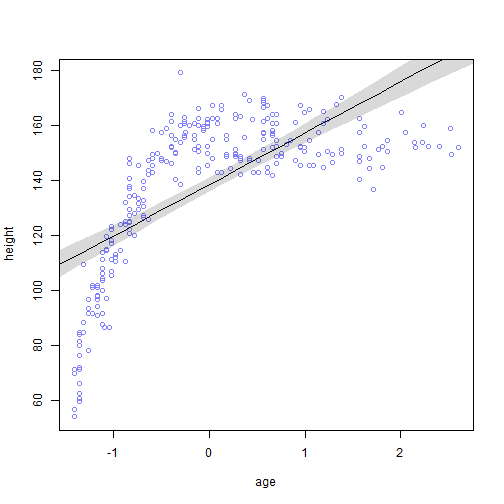age_plot(m2, d1, d.predict)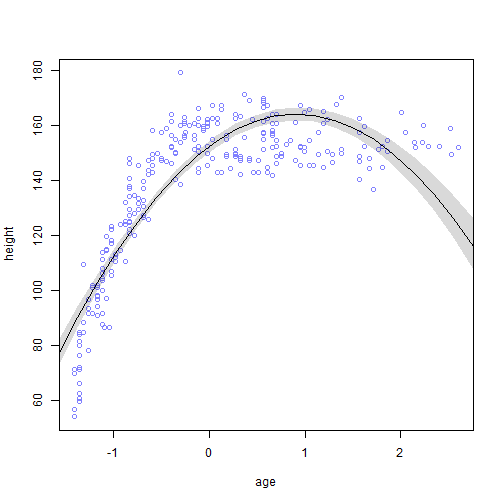age_plot(m3, d1, d.predict)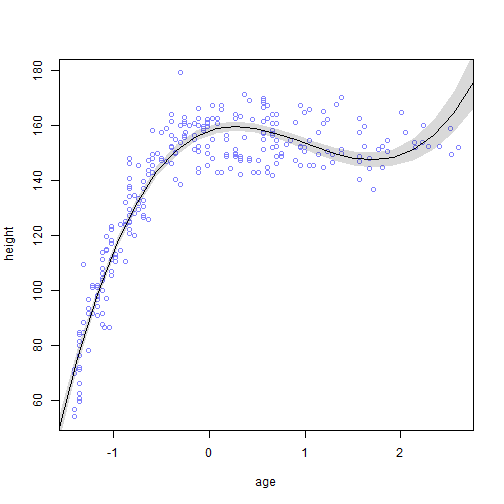age_plot(m4, d1, d.predict)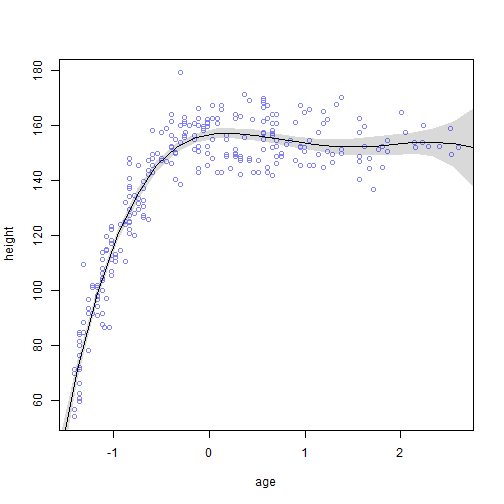age_plot(m5, d1, d.predict)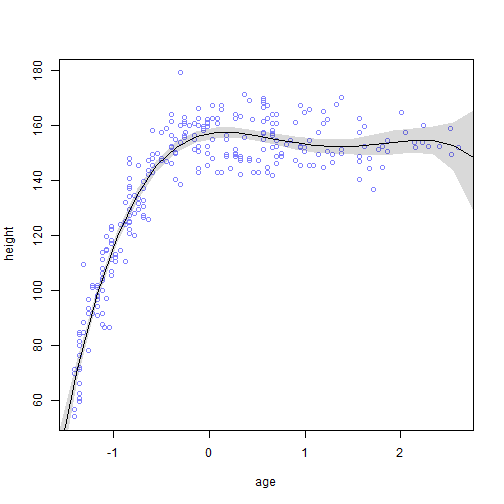age_plot(m6, d1, d.predict)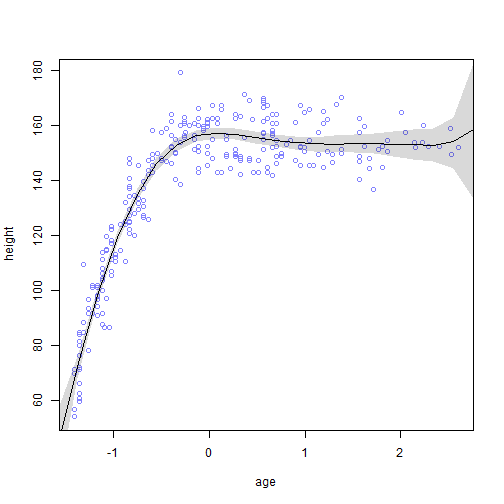The predictions differ pretty dramatically across models. $$\mathcal{M}_1$$ is underfit and only makes good predictions when age is around -1 and 1. $$\mathcal{M}_2$$ does a better job but makes poor predictions below -1.5 and above 1.5. $$\mathcal{M}_3$$ does a better job still but introduces an unlikely upward tick after around 2.0. $$\mathcal{M}_4$$ does the best job of all, but has a wide fanning out of its interval after around 2.0. $$\mathcal{M}_5$$ and $$\mathcal{M}_6$$ are similar to $$\mathcal{M}_4$$ for the majority of the age range, until around 2.5 when they both fan out even worse around either a down or up-tick.

### Practice Question 6H3

Now also plot the model averaged predictions, across all models. In what ways do the averaged predictions differ from the predictions of the model with the lowest WAIC value?

We can use the ensemble() function and reuse the d.predict data frame (page 204).

# Plot the lowest WAIC model with dashed lines
pred.m4 <- link(m4, data = d.predict, refresh = 0)
mu <- apply(pred.m4, 2, mean)
mu.PI <- apply(pred.m4, 2, PI, prob = 0.97)
plot(height ~ age, d1, col = rangi2)
lines(age.seq, mu, lty = 2)
lines(age.seq, mu.PI[1, ], lty = 2)
lines(age.seq, mu.PI[2, ], lty = 2)
# Calculate and plot model averaged predictions
height.ensemble <- ensemble(m1, m2, m3, m4, m5, m6, data = d.predict, refresh = 0)
mu <- apply(height.ensemble$link, 2, mean) mu.PI <- apply(height.ensemble$link, 2, PI, prob = 0.97)
lines(age.seq, mu)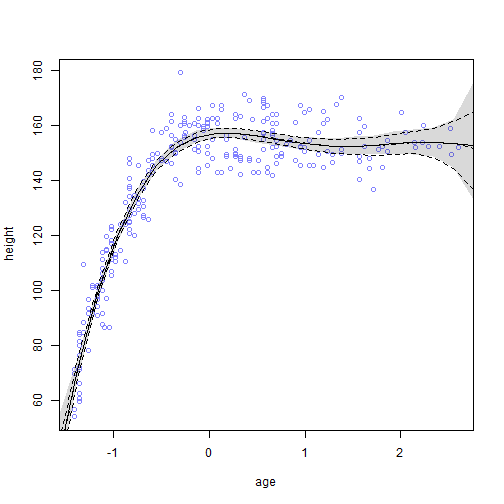The averaged predictions only differ from the lowest WAIC model (i.e., $$\mathcal{M}_4$$) by showing wider confidence intervals at the extreme ends of the age range. It is thus more conservative and communicates the uncertainty in this age range more clearly.

### Practice Question 6H4

Compute the test-sample deviance for each model. This means calculating deviance, but using the data in d2 now. You can compute the log-likelihood of the height data with:

sum(dnorm(d2$height, mu, sigma, log = TRUE)) where mu is a vector of predicted means (based upon age values and MAP parameters) and sigma is the MAP standard deviation. We can use the approach on page 183 to compute the test-sample deviance. The important intuitions here are that we need to calculate mu for each observation in d2 and then multiply the resulting log-likelihood by -2. # M1 theta <- coef(m1) (m1.d <- -2 * sum( dnorm( x = d2$height,
mean = theta + theta*d2$age, sd = theta, log = TRUE ) ))  ##  2421.868  # M2 theta <- coef(m2) (m2.d <- -2 * sum( dnorm( x = d2$height,
mean = theta + theta*d2$age + theta*d2$age^2,
sd = theta,
log = TRUE
)
))

##  2137.56

# M3
theta <- coef(m3)
(m3.d <- -2 * sum(
dnorm(
x = d2$height, mean = theta + theta*d2$age + theta*d2$age^2 + theta*d2$age^3,
sd = theta,
log = TRUE
)
))

##  1932.346

# M4
theta <- coef(m4)
(m4.d <- -2 * sum(
dnorm(
x = d2$height, mean = theta + theta*d2$age + theta*d2$age^2 + theta*d2$age^3 + theta*d2$age^4, sd = theta, log = TRUE ) ))  ##  1876.83  # M5 theta <- coef(m5) (m5.d <- -2 * sum( dnorm( x = d2$height,
mean = theta + theta*d2$age + theta*d2$age^2 + theta*d2$age^3 + theta*d2$age^4 +
theta*d2$age^5, sd = theta, log = TRUE ) ))  ##  1878.554  # M6 theta <- coef(m6) (m6.d <- -2 * sum( dnorm( x = d2$height,
mean = theta + theta*d2$age + theta*d2$age^2 + theta*d2$age^3 + theta*d2$age^4 +
theta*d2$age^5 + theta*d2$age^6,
sd = theta,
log = TRUE
)
))

##  1877.788


### Practice Question 6H5

Compare the deviances from 6H4 to the WAIC values. It might be easier to compare if you subtract the smallest value in each list from the others. For example, subtract the minimum WAIC from all of the WAIC values so that the best WAIC is normalized to zero. Which model makes the best out-of-sample predictions in this case? Does WAIC do a good job of estimating the test deviance?

Let’s begin by collecting the deviance and WAIC values in a data frame.

model.stats <- data.frame(
Model = c("M1", "M2", "M3", "M4", "M5", "M6"),
Deviance = c(m1.d, m2.d, m3.d, m4.d, m5.d, m6.d),
WAIC = c(WAIC(m1, refresh = 0), WAIC(m2, refresh = 0), WAIC(m3, refresh = 0),
WAIC(m4, refresh = 0), WAIC(m5, refresh = 0), WAIC(m6, refresh = 0))
)
model.stats$Deviance.C <- model.stats$Deviance - min(model.stats$Deviance) model.stats$WAIC.C <- model.stats$WAIC - min(model.stats$WAIC)
model.stats

##   Model Deviance     WAIC  Deviance.C     WAIC.C
## 1    M1 2421.868 2395.402 545.0372266 469.070460
## 2    M2 2137.560 2150.128 260.7297604 223.795917
## 3    M3 1932.346 1953.196  55.5152180  26.863549
## 4    M4 1876.830 1926.332   0.0000000   0.000000
## 5    M5 1878.554 1927.757   1.7240836   1.425026
## 6    M6 1877.788 1928.429   0.9573314   2.096813


$$\mathcal{M}_4$$ makes the best out-of-sample predictions as well as in-sample predictions. The ranking of models is also very similar by both WAIC and out-of-sample deviance. The only differences in ranking were swapping $$\mathcal{M}_5$$ and $$\mathcal{M}_6$$, although both were similar in magnitude. In this case, WAIC did a good job of estimating test deviance, although it tended to underestimate deviance for poorly fitting models. It’s also worth noting that we assigned observations to train and test sets randomly, which increases the likelihood that they are representative of one another.

### Practice Question 6H6

Consider the following model.

\begin{aligned}
h_i &\sim \mathrm{Normal}(\mu_i, \sigma) \\
\mu_i &= \alpha + \beta_1 x_i + \beta_2 x_i^2 + \beta_3 x_i^3 + \beta_4 x_i^4 + \beta_5 x_i^5 + \beta_6 x_i^6 \\
\beta_1 &\sim \mathrm{Normal}(0, 5) \\
\beta_2 &\sim \mathrm{Normal}(0, 5) \\
\beta_3 &\sim \mathrm{Normal}(0, 5) \\
\beta_4 &\sim \mathrm{Normal}(0, 5) \\
\beta_5 &\sim \mathrm{Normal}(0, 5) \\
\beta_6 &\sim \mathrm{Normal}(0, 5) \\
\end{aligned}

and assume flat (or nearly flat) priors on $$\alpha$$ and $$\sigma$$. This model contains more strongly regularizing priors on the coefficients.

First, fit this model to the data in d1. Report the MAP estimates and plot the implied predictions. Then compute the out-of-sample deviance using the data in d2, using MAP estimates from the model fit to d1 only. How does this model, using regularizing priors, compare to the best WAIC model from earlier? How do you interpret this result?

First, let’s calculate and report the MAP estimates.

m <- map(
alist(
height ~ dnorm(mu, sigma),
mu <- a + b1*age + b2*I(age^2) + b3*I(age^3) + b4*I(age^4) + b5*I(age^5) + b6*I(age^6),
a ~ dunif(50, 200),
b1 ~ dnorm(0, 5),
b2 ~ dnorm(0, 5),
b3 ~ dnorm(0, 5),
b4 ~ dnorm(0, 5),
b5 ~ dnorm(0, 5),
b6 ~ dnorm(0, 5),
sigma ~ dunif(0, 100)
),
data = d1
)
precis(m)

##         Mean StdDev   5.5%  94.5%
## a     155.86   0.88 154.46 157.26
## b1      5.97   1.81   3.08   8.86
## b2    -16.60   2.13 -20.01 -13.20
## b3     12.09   2.72   7.75  16.44
## b4     -3.51   1.16  -5.37  -1.65
## b5      0.23   1.04  -1.43   1.89
## b6      0.05   0.33  -0.48   0.58
## sigma   8.20   0.36   7.63   8.77


Next, let’s plot the implied predictions using the age_plot() function we created earlier.

age_plot(m, d1, d.predict)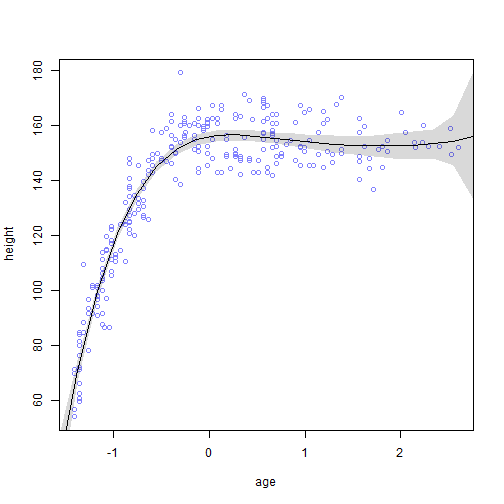Finally, let’s compute the out-of-sample deviance using the data in d2.

theta <- coef(m)
(m.d <- -2 * sum(
dnorm(
x = d2$height, mean = theta + theta*d2$age + theta*d2$age^2 + theta*d2$age^3 + theta*d2$age^4 + theta*d2$age^5 + theta*d2\$age^6,
sd = theta,
log = TRUE
)
))

##  1875.434

m.d - m4.d

##  -1.396751


This out-of-sample deviance estimate is slightly better than that of $$\mathcal{M}_4$$ (i.e., the best WAIC model from earlier). I interpret this result as an example of regularizing priors allowing the model to learn some, but not too much, from the data. For examples, this model includes fifth and sixth order polynomial terms, but their estimates are very imprecise in this case (more so than in $$\mathcal{M}_6$$).

### Session Info

sessionInfo()

## R version 3.5.1 (2018-07-02)
## Platform: x86_64-w64-mingw32/x64 (64-bit)
## Running under: Windows >= 8 x64 (build 9200)
##
## Matrix products: default
##
## locale:
##  LC_COLLATE=English_United States.1252
##  LC_CTYPE=English_United States.1252
##  LC_MONETARY=English_United States.1252
##  LC_NUMERIC=C
##  LC_TIME=English_United States.1252
##
## attached base packages:
##  parallel  stats     graphics  grDevices utils     datasets  methods
##  base
##
## other attached packages:
##  RWordPress_0.2-3     knitr_1.21           rethinking_1.59
##
## loaded via a namespace (and not attached):
##   Rcpp_1.0.0         mvtnorm_1.0-8      lattice_0.20-35
##   prettyunits_1.0.2  ps_1.3.0           assertthat_0.2.0
##   rprojroot_1.3-2    digest_0.6.18      mime_0.6
##  R6_2.3.0           plyr_1.8.4         backports_1.1.3
##  stats4_3.5.1       evaluate_0.12      coda_0.19-2
##  highr_0.7          pillar_1.3.1       rlang_0.3.0.1
##  curl_3.2           lazyeval_0.2.1     rstudioapi_0.8
##  callr_3.1.1        rmarkdown_1.11     desc_1.2.0
##  devtools_2.0.1     stringr_1.3.1      loo_2.0.0
##  RCurl_1.95-4.11    munsell_0.5.0      compiler_3.5.1
##  xfun_0.4           pkgconfig_2.0.2    base64enc_0.1-3
##  pkgbuild_1.0.2     htmltools_0.3.6    tidyselect_0.2.5
##  tibble_1.4.2       gridExtra_2.3      matrixStats_0.54.0
##  XML_3.98-1.16      crayon_1.3.4       dplyr_0.7.8
##  withr_2.1.2        bitops_1.0-6       MASS_7.3-50
##  grid_3.5.1         jsonlite_1.6       gtable_0.2.0
##  magrittr_1.5       scales_1.0.0       cli_1.0.1
##  stringi_1.2.4      fs_1.2.6           remotes_2.0.2
##  bindrcpp_0.2.2     testthat_2.0.1     tools_3.5.1
##  glue_1.3.0         markdown_0.9       purrr_0.2.5